CBSE Class 12 Sample Paper for 2019 Boards

Class 12
Solutions of Sample Papers and Past Year Papers - for Class 12 Boards

Question 27 (OR 2 nd question)

Show that the line of intersection of the planes x + 2y + 3z = 8 and 2x + 3y + 4z = 11 is coplanar with the line (x + 1)/1 = (y + 1)/2 = (z + 1)/3 . Also find the equation of the plane containing them.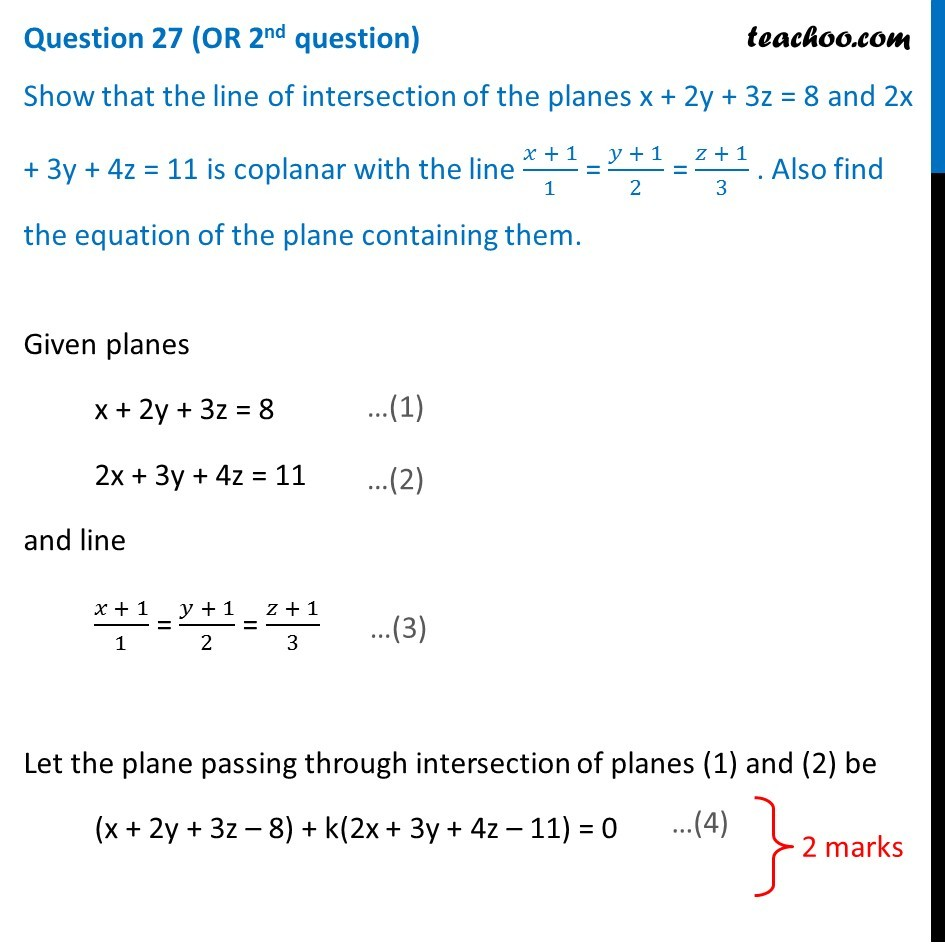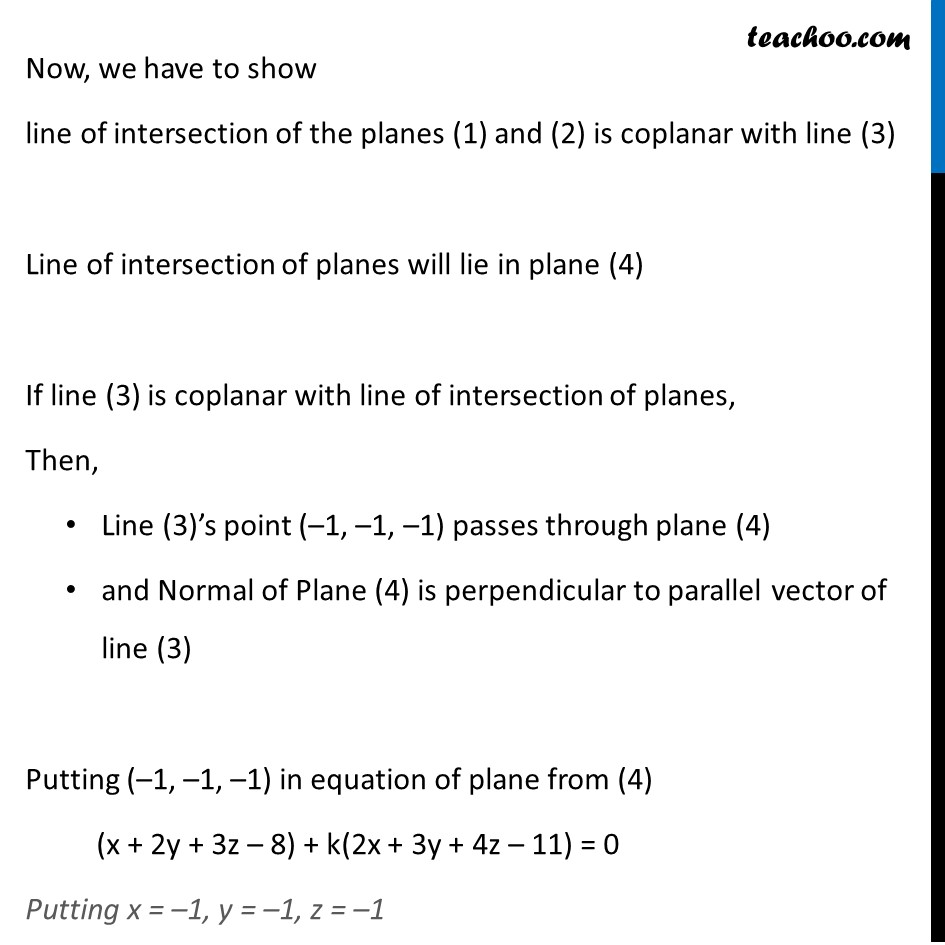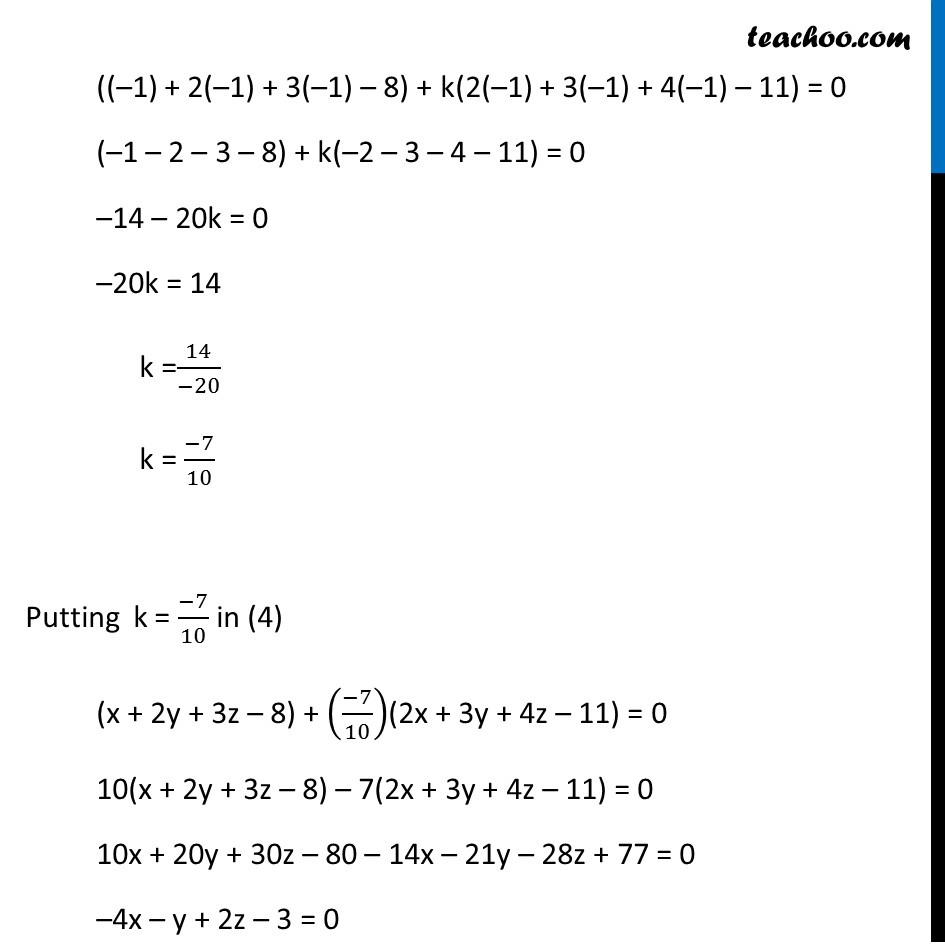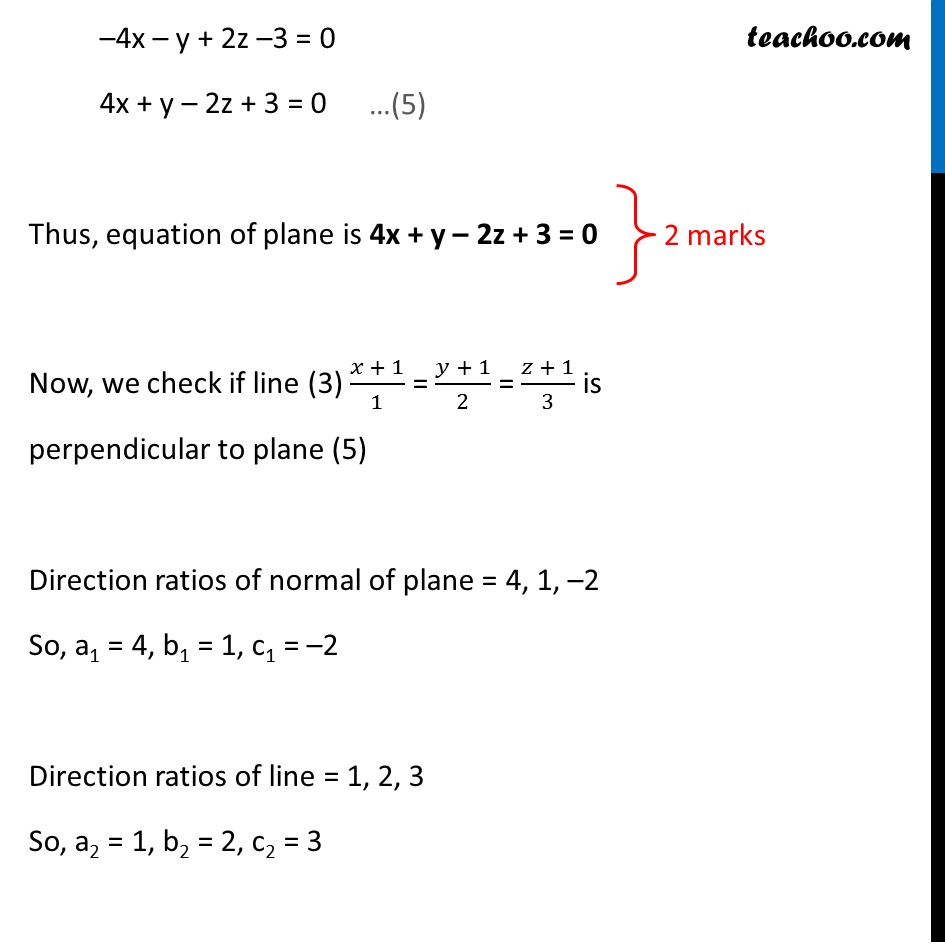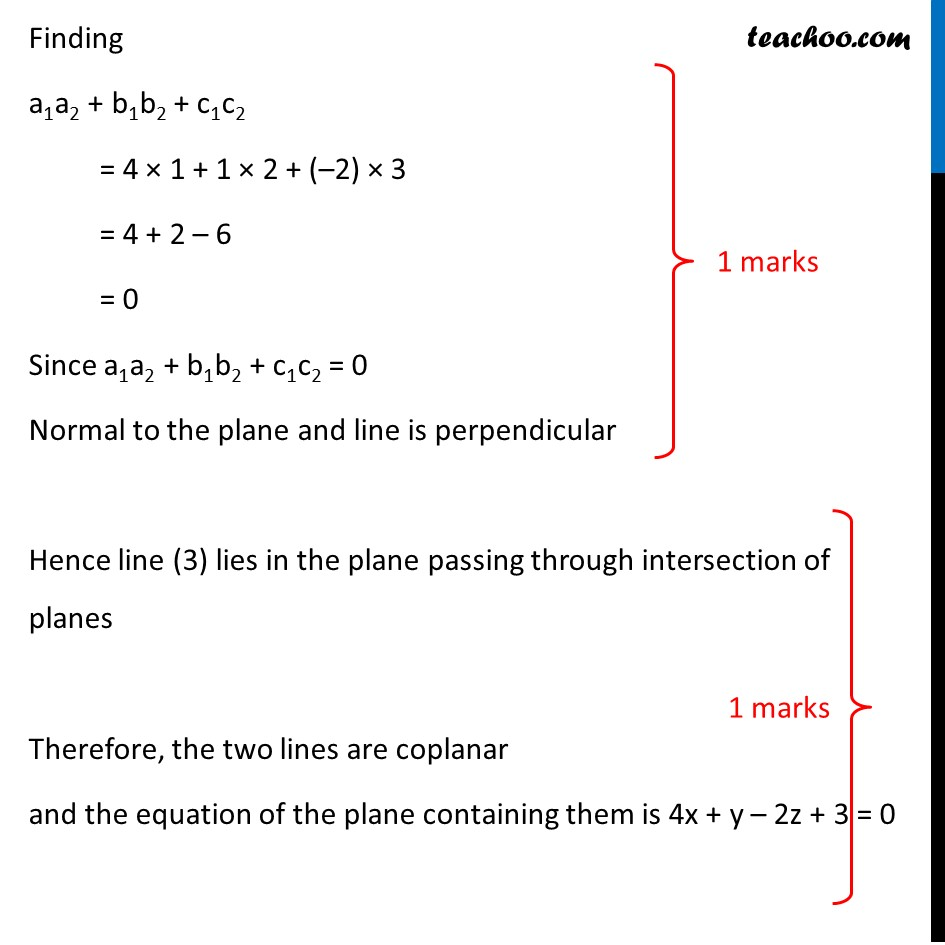Learn in your speed, with individual attention - Teachoo Maths 1-on-1 Class

### Transcript

Question 27 (OR 2nd question) Show that the line of intersection of the planes x + 2y + 3z = 8 and 2x + 3y + 4z = 11 is coplanar with the line (𝑥 + 1)/1 = (𝑦 + 1)/2 = (𝑧 + 1)/3 . Also find the equation of the plane containing them. Given planes x + 2y + 3z = 8 2x + 3y + 4z = 11 and line (𝑥 + 1)/1 = (𝑦 + 1)/2 = (𝑧 + 1)/3 Let the plane passing through intersection of planes (1) and (2) be (x + 2y + 3z – 8) + k(2x + 3y + 4z – 11) = 0 Now, we have to show line of intersection of the planes (1) and (2) is coplanar with line (3) Line of intersection of planes will lie in plane (4) If line (3) is coplanar with line of intersection of planes, Then, Line (3)’s point (–1, –1, –1) passes through plane (4) and Normal of Plane (4) is perpendicular to parallel vector of line (3) Putting (–1, –1, –1) in equation of plane from (4) (x + 2y + 3z – 8) + k(2x + 3y + 4z – 11) = 0 Putting x = –1, y = –1, z = –1 ((–1) + 2(–1) + 3(–1) – 8) + k(2(–1) + 3(–1) + 4(–1) – 11) = 0 (–1 – 2 – 3 – 8) + k(–2 – 3 – 4 – 11) = 0 –14 – 20k = 0 –20k = 14 k =14/(−20) k = (−7)/10 Putting k = (−7)/10 in (4) (x + 2y + 3z – 8) + ((−7)/10)(2x + 3y + 4z – 11) = 0 10(x + 2y + 3z – 8) – 7(2x + 3y + 4z – 11) = 0 10x + 20y + 30z – 80 – 14x – 21y – 28z + 77 = 0 –4x – y + 2z – 3 = 0 –4x – y + 2z –3 = 0 4x + y – 2z + 3 = 0 Thus, equation of plane is 4x + y – 2z + 3 = 0 Now, we check if line (3) (𝑥 + 1)/1 = (𝑦 + 1)/2 = (𝑧 + 1)/3 is perpendicular to plane (5) Direction ratios of normal of plane = 4, 1, –2 So, a1 = 4, b1 = 1, c1 = –2 Direction ratios of line = 1, 2, 3 So, a2 = 1, b2 = 2, c2 = 3 Finding a1a2 + b1b2 + c1c2 = 4 × 1 + 1 × 2 + (–2) × 3 = 4 + 2 – 6 = 0 Since a1a2 + b1b2 + c1c2 = 0 Normal to the plane and line is perpendicular Hence line (3) lies in the plane passing through intersection of planes Therefore, the two lines are coplanar and the equation of the plane containing them is 4x + y – 2z + 3 = 0